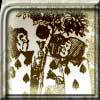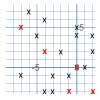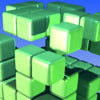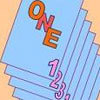# Resources tagged with: Visualising

Filter by: Content type:
Age range:
Challenge level:

### There are 180 results

Broad Topics > Thinking Mathematically > Visualising### AMGM

##### Age 14 to 16 Challenge Level:

Can you use the diagram to prove the AM-GM inequality?### The Triangle Game

##### Age 11 to 16 Challenge Level:

Can you discover whether this is a fair game?### Partly Painted Cube

##### Age 14 to 16 Challenge Level:

Jo made a cube from some smaller cubes, painted some of the faces of the large cube, and then took it apart again. 45 small cubes had no paint on them at all. How many small cubes did Jo use?### Christmas Chocolates

##### Age 11 to 14 Challenge Level:

How could Penny, Tom and Matthew work out how many chocolates there are in different sized boxes?### Tourism

##### Age 11 to 14 Challenge Level:

If you can copy a network without lifting your pen off the paper and without drawing any line twice, then it is traversable. Decide which of these diagrams are traversable.### Clocked

##### Age 11 to 14 Challenge Level:

Is it possible to rearrange the numbers 1,2......12 around a clock face in such a way that every two numbers in adjacent positions differ by any of 3, 4 or 5 hours?### Cubes Within Cubes

##### Age 7 to 14 Challenge Level:

We start with one yellow cube and build around it to make a 3x3x3 cube with red cubes. Then we build around that red cube with blue cubes and so on. How many cubes of each colour have we used?### Flight of the Flibbins

##### Age 11 to 14 Challenge Level:

Blue Flibbins are so jealous of their red partners that they will not leave them on their own with any other bue Flibbin. What is the quickest way of getting the five pairs of Flibbins safely to. . . .### Tilting Triangles

##### Age 14 to 16 Challenge Level:

A right-angled isosceles triangle is rotated about the centre point of a square. What can you say about the area of the part of the square covered by the triangle as it rotates?### Hypotenuse Lattice Points

##### Age 14 to 16 Challenge Level:

The triangle OMN has vertices on the axes with whole number co-ordinates. How many points with whole number coordinates are there on the hypotenuse MN?### Painting Cubes

##### Age 11 to 14 Challenge Level:

Imagine you have six different colours of paint. You paint a cube using a different colour for each of the six faces. How many different cubes can be painted using the same set of six colours?### Convex Polygons

##### Age 11 to 14 Challenge Level:

Show that among the interior angles of a convex polygon there cannot be more than three acute angles.### All in the Mind

##### Age 11 to 14 Challenge Level:

Imagine you are suspending a cube from one vertex and allowing it to hang freely. What shape does the surface of the water make around the cube?### Coloured Edges

##### Age 11 to 14 Challenge Level:

The whole set of tiles is used to make a square. This has a green and blue border. There are no green or blue tiles anywhere in the square except on this border. How many tiles are there in the set?### Paving Paths

##### Age 11 to 14 Challenge Level:

How many different ways can I lay 10 paving slabs, each 2 foot by 1 foot, to make a path 2 foot wide and 10 foot long from my back door into my garden, without cutting any of the paving slabs?### A Tilted Square

##### Age 14 to 16 Challenge Level:

The opposite vertices of a square have coordinates (a,b) and (c,d). What are the coordinates of the other vertices?### Jam

##### Age 14 to 16 Challenge Level:

To avoid losing think of another very well known game where the patterns of play are similar.### How Many Dice?

##### Age 11 to 14 Challenge Level:

A standard die has the numbers 1, 2 and 3 are opposite 6, 5 and 4 respectively so that opposite faces add to 7? If you make standard dice by writing 1, 2, 3, 4, 5, 6 on blank cubes you will find. . . .### Cube Paths

##### Age 11 to 14 Challenge Level:

Given a 2 by 2 by 2 skeletal cube with one route `down' the cube. How many routes are there from A to B?### Concrete Wheel

##### Age 11 to 14 Challenge Level:

A huge wheel is rolling past your window. What do you see?### Natural Sum

##### Age 14 to 16 Challenge Level:

The picture illustrates the sum 1 + 2 + 3 + 4 = (4 x 5)/2. Prove the general formula for the sum of the first n natural numbers and the formula for the sum of the cubes of the first n natural. . . .### Dotty Triangles

##### Age 11 to 14 Challenge Level:

Imagine an infinitely large sheet of square dotty paper on which you can draw triangles of any size you wish (providing each vertex is on a dot). What areas is it/is it not possible to draw?### Rati-o

##### Age 11 to 14 Challenge Level:

Points P, Q, R and S each divide the sides AB, BC, CD and DA respectively in the ratio of 2 : 1. Join the points. What is the area of the parallelogram PQRS in relation to the original rectangle?### Königsberg

##### Age 11 to 14 Challenge Level:

Can you cross each of the seven bridges that join the north and south of the river to the two islands, once and once only, without retracing your steps?### Something in Common

##### Age 14 to 16 Challenge Level:

A square of area 3 square units cannot be drawn on a 2D grid so that each of its vertices have integer coordinates, but can it be drawn on a 3D grid? Investigate squares that can be drawn.### The Farmers' Field Boundary

##### Age 11 to 14 Challenge Level:

The farmers want to redraw their field boundary but keep the area the same. Can you advise them?### Tower of Hanoi

##### Age 11 to 14 Challenge Level:

The Tower of Hanoi is an ancient mathematical challenge. Working on the building blocks may help you to explain the patterns you notice.### Steel Cables

##### Age 14 to 16 Challenge Level:

Some students have been working out the number of strands needed for different sizes of cable. Can you make sense of their solutions?### Eight Hidden Squares

##### Age 7 to 14 Challenge Level:

On the graph there are 28 marked points. These points all mark the vertices (corners) of eight hidden squares. Can you find the eight hidden squares?##### Age 11 to 14 Challenge Level:

Can you mark 4 points on a flat surface so that there are only two different distances between them?### Cubes Within Cubes Revisited

##### Age 11 to 14 Challenge Level:

Imagine starting with one yellow cube and covering it all over with a single layer of red cubes, and then covering that cube with a layer of blue cubes. How many red and blue cubes would you need?### Ten Hidden Squares

##### Age 7 to 14 Challenge Level:

These points all mark the vertices (corners) of ten hidden squares. Can you find the 10 hidden squares?### Seven Squares - Group-worthy Task

##### Age 11 to 14 Challenge Level:

Choose a couple of the sequences. Try to picture how to make the next, and the next, and the next... Can you describe your reasoning?### Building Tetrahedra

##### Age 14 to 16 Challenge Level:

Can you make a tetrahedron whose faces all have the same perimeter?### Square It

##### Age 11 to 16 Challenge Level:

Players take it in turns to choose a dot on the grid. The winner is the first to have four dots that can be joined to form a square.### Sliding Puzzle

##### Age 11 to 16 Challenge Level:

The aim of the game is to slide the green square from the top right hand corner to the bottom left hand corner in the least number of moves.### On the Edge

##### Age 11 to 14 Challenge Level:

If you move the tiles around, can you make squares with different coloured edges?### Jam

##### Age 14 to 16 Challenge Level:

A game for 2 players### Frogs

##### Age 11 to 14 Challenge Level:

How many moves does it take to swap over some red and blue frogs? Do you have a method?### Picturing Triangular Numbers

##### Age 11 to 14 Challenge Level:

Triangular numbers can be represented by a triangular array of squares. What do you notice about the sum of identical triangle numbers?### Framed

##### Age 11 to 14 Challenge Level:

Seven small rectangular pictures have one inch wide frames. The frames are removed and the pictures are fitted together like a jigsaw to make a rectangle of length 12 inches. Find the dimensions of. . . .### Picture Story

##### Age 14 to 16 Challenge Level:

Can you see how this picture illustrates the formula for the sum of the first six cube numbers?### Shear Magic

##### Age 11 to 14 Challenge Level:

Explore the area of families of parallelograms and triangles. Can you find rules to work out the areas?### Marbles in a Box

##### Age 11 to 16 Challenge Level:

How many winning lines can you make in a three-dimensional version of noughts and crosses?### The Perforated Cube

##### Age 14 to 16 Challenge Level:

A cube is made from smaller cubes, 5 by 5 by 5, then some of those cubes are removed. Can you make the specified shapes, and what is the most and least number of cubes required ?### Chess

##### Age 11 to 14 Challenge Level:

What would be the smallest number of moves needed to move a Knight from a chess set from one corner to the opposite corner of a 99 by 99 square board?### Squares in Rectangles

##### Age 11 to 14 Challenge Level:

A 2 by 3 rectangle contains 8 squares and a 3 by 4 rectangle contains 20 squares. What size rectangle(s) contain(s) exactly 100 squares? Can you find them all?### Proximity

##### Age 14 to 16 Challenge Level:

We are given a regular icosahedron having three red vertices. Show that it has a vertex that has at least two red neighbours.### Dissect

##### Age 11 to 14 Challenge Level:

What is the minimum number of squares a 13 by 13 square can be dissected into?### One Out One Under

##### Age 14 to 16 Challenge Level:

Imagine a stack of numbered cards with one on top. Discard the top, put the next card to the bottom and repeat continuously. Can you predict the last card?